### Home > A2C > Chapter 9 > Lesson 9.1.3 > Problem9-57

9-57.
1. For each of the following polynomial expressions, find the degree, list the coefficients, and then label them a0 through an. Refer to the example in the Lesson 9.1.3 Math Notes box above about polynomial notation. Homework Help ✎

1. 6x4 − 3x3 + 5x2 + x + 8

2. −5x3 + 10x2 + 8

3. x2 + x

4. x(x − 3)(x − 5)

5. x

6. 10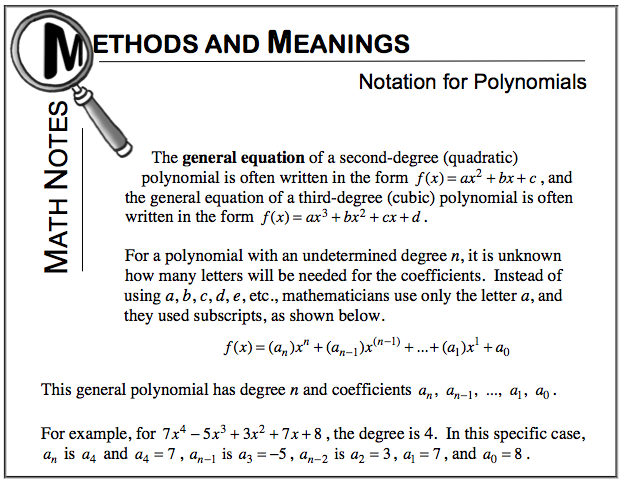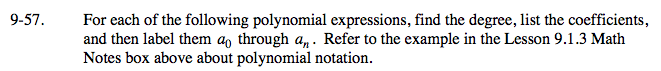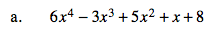Degree 4,
a4 = 6, a3 = −3, a2 = 5, a1 = 1, a0 = 8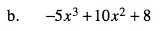Degree 3,
a3 = −5, a2 = 10, a1 = 1, a0 = 8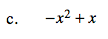See the Math Notes box.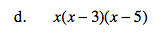Simplify first.

Degree 3,
a3 = 1, a2 = − 8, a1 = 15, a0 = 0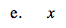See the Math Notes box.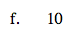See the Math Notes box.